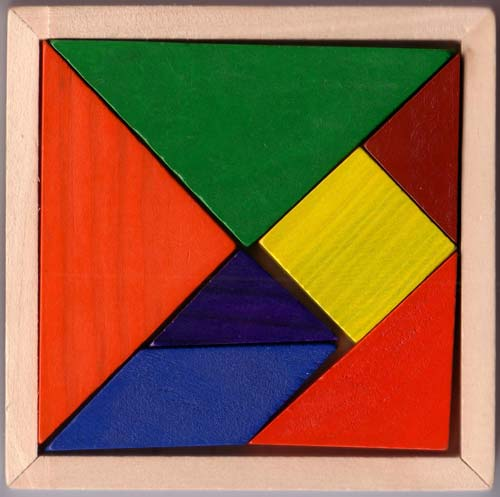A# geometry puzzlesOne of the attractions of puzzles involving shapes, especially dissection problems, is that they appeal to the eye and very often don't call for much ability in solving equations and the like. Anyone can try to assemble the pieces of a jigsaw, whether they be of a picture or of a geometric shape, so a mathematical game such Tangrams or the Soma Cube is within everyone's reach. On the other hand, some geometric puzzles call for a basic knowledge of more abstract fields such as algebra and calculus. They may also exploit our sometimes faulty intuition about how different quantities vary in one, two, and three dimensions and about how much information is needed to solve a problem.

As an example of faulty intuition, imagine that the Earth, taken to be a perfect sphere with a radius r of 6,378 km, is completely covered by a thin membrane. Now suppose that one square meter is added to the area of this membrane to form a larger sphere. By how much does the radius and the volume of this membrane increase? This can be worked out from the formulae for the volume of a sphere (V = (4/3)πr 3) and the area of a sphere (A = 4πr 2), respectively. It turns out that if the area of the cover is increased by 1 square meter, then the volume it contains is increased by about 3.25 million cubic meters. This seems like a huge amount. However, the new cover wouldn't be very high above the surface of the planet – only about 6 nanometers! As an example of problem that is both counterintuitive and seems to lack sufficient data for its solution see hole-through-a-sphere problem.